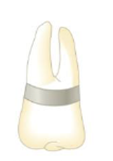# The hand in Figure P10.23 is stainless steel (coefficient of linear expansion = 17.3 × 10 −6 (°C) −1 ; Young’s modulus = 18 × 10 10 N/m 2 ). It is essentially circular with an initial mean radius of 5.0 mm, a height of 4.0 mm, and a thickness of 0.50 mm. If the band just fits snugly over the tooth when heated to a temperature of 80.0°C, what is the tension in the hand when it cools to a temperature of 37°C? Figure P10.23### College Physics

11th Edition
Raymond A. Serway + 1 other
Publisher: Cengage Learning
ISBN: 9781305952300### College Physics

11th Edition
Raymond A. Serway + 1 other
Publisher: Cengage Learning
ISBN: 9781305952300

#### Solutions

Chapter
Section
Chapter 10, Problem 23P
Textbook Problem

## The hand in Figure P10.23 is stainless steel (coefficient of linear expansion = 17.3 × 10−6(°C)−1; Young’s modulus = 18 × 1010 N/m2). It is essentially circular with an initial mean radius of 5.0 mm, a height of 4.0 mm, and a thickness of 0.50 mm. If the band just fits snugly over the tooth when heated to a temperature of 80.0°C, what is the tension in the hand when it cools to a temperature of 37°C?Figure P10.23

Expert Solution
To determine
The tension in the band.

### Explanation of Solution

Given Info: The length of the bridge is 1.29 km. The radius of the band is 5.0 mm. The height is 4.0 mm (h) and the thickness (t) is 0.50 mm. The temperature drops from T1=37.0οC to T2=80.0οC . The Young’s modulus (Y) for stainless steel is 18×1010Nm2 . The co-efficient of linear expansion ( α ) of stainless steel is 17.3×106/οC .

Formula to calculate the tension is,

F=AY(ΔLL0)       (I)

• A is the cross sectional area.

Formula to calculate the cross sectional area is,

A=ht       (II)

Formula to calculate the change in length is,

ΔLL0=α(T2T1)       (III)

From Equations (I) , (II) and (III),

F=htYα|(T2T1)|

Substitute 4.0 mm for h, 0.50 mm for t, 18×1010Nm2 for Y, 17.3×106/οC for α , 80.0οC for T2 and 37

### Want to see the full answer?

Check out a sample textbook solution.See solution

### Want to see this answer and more?

Bartleby provides explanations to thousands of textbook problems written by our experts, many with advanced degrees!

See solution